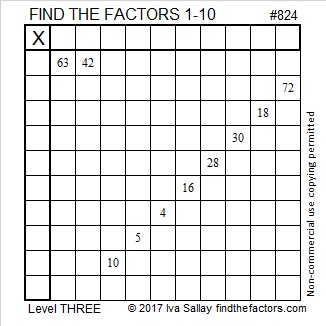# 824 and Level 3

824 is the sum of all the prime numbers from 61 all the way to 103, which just happens to be one of its prime factors!Print the puzzles or type the solution on this excel file: 10-factors-822-828

824 is a leg in a few Pythagorean triples:

• 618-824-1030 because that is 206 times (3-4-5)
• 824-1545-1751 because that is 103 times (8-15-17)
• 824-10593-10625 because 2(103)(4) = 824
• 824-21210-21226 because 105² – 101² = 824
• 824-42432-42440 because 2(206)(2) = 824
• 824-84870-84874 because 207² – 205² = 824
• Primitive 824-169743-169745 because 2(412)(1) = 824

Five of those triples were derived directly from 824’s factor pairs.

Two of the triples were derived indirectly:

• What is (105+101)/2, (105-101)/2?
• Also, what is (207+205)/2, (207+205)/2?

The answer to both questions is a factor pair of 824.

You can read more about finding Pythagorean triples for numbers that are divisible by 4 here.

• 824 is a composite number.
• Prime factorization: 824 = 2 × 2 × 2 × 103, which can be written 824 = 2³ × 103
• The exponents in the prime factorization are 3 and 1. Adding one to each and multiplying we get (3 + 1)(1 + 1) = 4 × 2 = 8. Therefore 824 has exactly 8 factors.
• Factors of 824: 1, 2, 4, 8, 103, 206, 412, 824
• Factor pairs: 824 = 1 × 824, 2 × 412, 4 × 206, or 8 × 103
• Taking the factor pair with the largest square number factor, we get √824 = (√4)(√206) = 2√206 ≈ 28.7054This site uses Akismet to reduce spam. Learn how your comment data is processed.